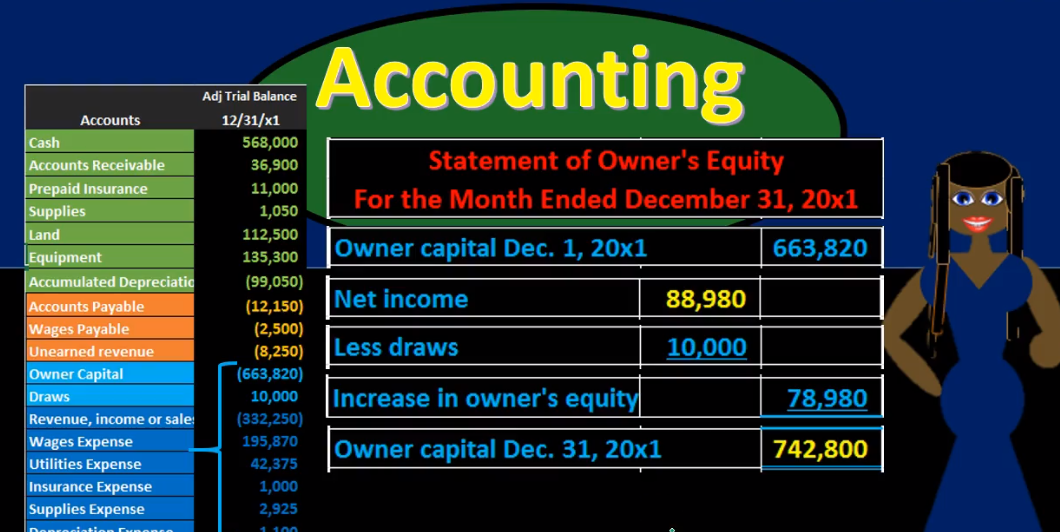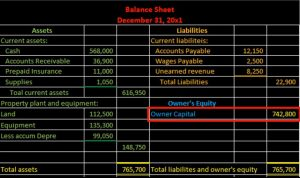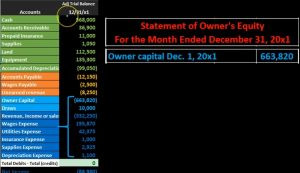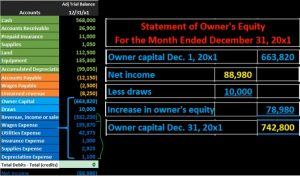## Statement of Equity From Trial Balance 17

More in this presentation we will take a look at the statement of owner’s equity and see how to construct the statement of owner’s equity from the trial balance. When looking at the trial balance, we can see the accounts will be in order with the assets and then the liabilities, then the equity and then the revenue and expenses. The equity accounts being broken out here of owner capital and draws. But it’s a little deceiving to break out this equity section. Because the trial balance really is showing both a point in time the balance sheet account permanent accounts up top and timing accounts which are going to be the revenue accounts down below. When we think about the point in time for total equity as a whole. We’re really considering the entire blue area here. This is one of the most confusing concepts to really know when you’re looking at these financial statements.

00:49

And it’s really the timing concept that can be something that’s difficult in general and once we get a good understanding of this helps a lot in many different areas. When considering the equities section then we’re considering really all of these blue accounts, we’re going to put all these together, we already grouped some of them together on the income statement. And now we’re going to complete the process. We now want to consider how the statement of equity will fit in to the balance sheet to the double entry accounting system expressed through the accounting equation have assets equal liabilities and equity, which is represented on the balance sheet. And of course, we’re thinking here of the equity section. So we have this number in the equity section. And this is for a sole proprietor if it was a partnership, it would be partnership equity, if it’s if it’s a corporation, it’ll include retained earnings or shareholders equity will be involved here.

01:41

And the same type of concepts will be true in that whatever the equity section is here named in terms of the type of entity it is, we’re going to want to get more detail in terms of the timing of it. This is what it’s going to be as of the end of the time period, and we want to break out the detail of it. That includes mainly the income tax. statement, which we’ve talked about prior, and then the statement of owner’s equity, which will tie in the income statement, and take care of any other type of accounts such as draws. When considering this relationship to the trial balance, we think of the income statement here. If we look at the income statement, it’s picking up a lot of these blue accounts. That’s what we’re talking about. That’s what we want to pick up. The income statement is picking up a lot of these blue accounts here, but they’re not picking up all of them. So we crunched these group blue accounts into what we call net income, bottom line number of the income statement.02:33

Now we just need to pick up the last couple numbers here, which is the beginning capital account and the draws. So that’s what we’ll do in the statement of equity. We’re going to say okay, we’re going to use this number that was created on the income statement is 88 980, this net income, and we’ll add to that the beginning balance, and we’ll take into account the draws. So now we’re going to construct the statement of owner’s equity note that we’re going to have for the month ended December 31 2000 x one similar to the income statement, a timing statement, rather than a point in time as is expressed on the balance sheet. So the balance sheet will just have the one date as of this point in time. And then the income statement, the statement of equity will have this date range, typically expressed in this format for the month ended, in this case, December 31, which means December 1 through December 31. As we create this, then we’re looking at this entire portion all the blue accounts, that’s what we want to pick up. So we’ve got the owner capital.

03:36

Note that we’re going to include a date here, owner capital December 1, and that’s the beginning of the month at the beginning of the month. And we picked up this 663 820 from here, so this 663 a 20. It’s a credit here, we’re not going to put debits and credits when we pull it over to this side. We’re just going to be using plus and minus. We are going to put it in an outer column here, not because it’s a credit Because if we’re going to have subcategories to add and subtract, and note, the date here is December 31, really deceiving, because when you see it here, the date on this entire trial balance is 1231, the end of the time period, the end of the year. And we’re saying here, we’ve picked up this number from the trial balance, which says it’s as of 1231 and labeling it December 1. And that’s because you know, this, this label on the trial balance isn’t exactly accurate entirely, because it’s representing both timing accounts and permanent accounts, as if they’re all permanent with one day, but they’re not because these accounts down here represent time and accounts.

04:43

They represent what happened from the first to the end of the month. So this account right here is actually the owner capital as of the end of the beginning of the time period. And if there’s any activities such as draws, or investments, it would also be in there. How would we know that We’d have to take a look at the GL, see if there’s any kind of transactions in there. Typically, if there’s no investments, this number will be the same. And it will not have changed over the time period, because we don’t post things typically to the owner capital, unless it’s an investment, then we’re going to have detail that will be included, which will be everything underneath it. In essence, we’re going to be crunching all these blue numbers into one number, giving us the true point in time as of 1231 x one, our total equity. Next thing we’re going to have is going to be the net income. Where’s the net income going to come from the income statement. So the income statement has been created, we have net income, and we pulled that over. In essence, what we did is we just took all these numbers here.

05:45

And we broke them out into the income statement, meaning we took the revenue minus the expenses given us the one number 888, nine ad that’s given in net income, so we have the ad 898 Hear crunching all these numbers into here. So we just basically took care of, of all these numbers and found a home for them, then we have the draws, there’s going to be the one account that has not been used on the income statement, it is a a debit here, which is actually reducing the total equity. Over here, we’re going to put it in and we’re going to say less to say that it’s going to be a subtraction here. Now this can be a little confusing to know which way the signs are going how to adjust the signs to a plus and minus format. Over here, we know that the credits are going to be winning. So we have a credit balance minus the debit balance, plus the credit balance minus all the debits will give us our ending number. Over here, we just have to reflect that same thing.06:44

We got to say, well, the owners capital as a credit, it’s going to be winning. Here’s the credit balance, we’re going to add to its net income. And then we’re going to subtract draws, we’re going to reduce it by draws, because of course draws represent something that’s been pulled out of the company. To the owner, therefore, the amount owed to the owner, as of the end of the time period has then gone down, we could represent that with a sign like a negative sign or brackets. But oftentimes, it’ll be expressed with words, we’ll just say less here to express a subtraction problem, then we’re going to pull that out to the outer columns, we have an increase in owner’s equity, that’s really kind of a net increase crease, it’s typically just called an increase, but what we’re doing is sub categorizing these two numbers are saying, here’s what happened over the time period crunched up into one number, we had net income, bringing the equity up, then we had draws bringing it down, subtracting those two gives us the increase in the equity. So here’s the increase in the equity we’re breaking out into the outer column, not because of debits and credits, but because of a subcategory type of function, where we have the two items here being calculated together in the outer column there.07:55

Then we’re finally going to get to the owner capital as of December 31 2000. x one. So here’s the indeed owner capital. Once again, we’re not jumping back and forth between different columns, we’re not going to calculate in here and then calculated in here, we’re just calculating the outer column here. So we’ve got the 663 820 minus 278 980, bringing us to that 740 to 800. For our Indian number, the Cindy number is what we will find on the balance sheet. The Cindy number is how the statement of equity will fit into the double entry accounting system represented by the accounting equation. This being part of course, the equity section assets equal liabilities plus equity. So if you consider the relationships now between the financial statements, we know that the income statement is used to create the statement of equity, and we know that the statement of equity balance is used to create the equity portion of the balance sheet